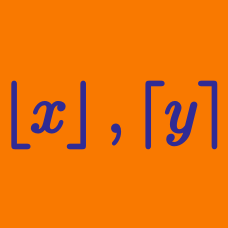Algebra

# Floor and Ceiling Functions: Level 5 Challenges

Find the number of positive integers $x$ which satisfiy

$\left\lfloor \dfrac {x}{99} \right\rfloor = \left\lfloor \dfrac {x}{101} \right\rfloor .$

$\left\lceil 1 \right\rceil + \left\lceil 1.7 \right\rceil + \left\lceil 2.4 \right\rceil + \left\lceil 3.1 \right\rceil+\ldots+\left\lceil 999.9 \right\rceil=?$

Given that $1\le x \le k,$ there are 2017 solutions to the equation $\large x^3-\left\lfloor x^3 \right\rfloor = \left( x - \lfloor x \rfloor \right)^3.$ The minimum value of $k$ is $a+\frac{b\sqrt{c}}{d},$ where $a, b, c, d$ are positive integers.

Given that $c$ is square-free and $b$ and $d$ are coprime, find the value of $a+b+c+d.$


Notation: $\lfloor \cdot \rfloor$ denotes the floor function.

Find

$\displaystyle\sum_{k=1}^{1995}\dfrac 1{f(k)},$

where $f(n)$ is the integer closest to $\sqrt{n}.$

If the range of positive $x$ satisfy the equation $\lceil x \lfloor x \rfloor \rceil + \lfloor x \lceil x \rceil \rfloor = 111$ is $\alpha \leq x \leq \beta$. What is the value of $8\alpha + 7 \beta$?

×